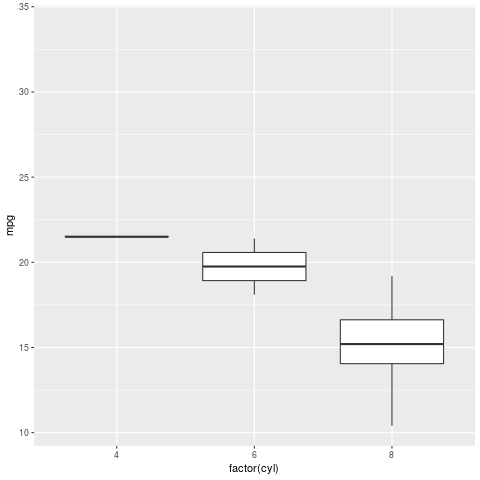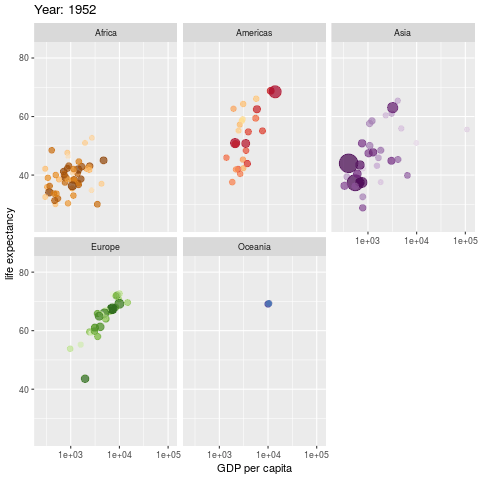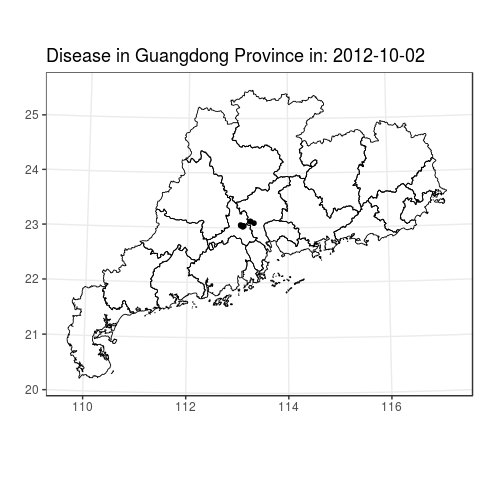# @写在这中秋前夜: 动起来

## 动画制作

### 程序包安装

gganimate程序包即可通过官网上下载,也可通过github(thomasp85)安装, 需要注意的是github版本一般会较早更新. 安装的具体代码如下:

• 官网途径(因更新版本,官网途径已经失效):
install.packages("gganimate")

• Github途径: 需要先下载devtools程序包,然后用install_github函数进行安装:
# install.packages('devtools')
devtools::install_github('thomasp85/gganimate')


### 主要函数

• transition_*(): 定义动画是根据哪个变量进行”动”.
• view_*(): 定义标尺的位置.
• shadow_*(): 影子?定义点相继出现的方式.
• enter_*()/exit_*(): 定义新数据产生和旧数据删除的方式.
• ease_aes(): 美观定义(如何让整个动画看起来更舒适).

(注意,此处只列出部分函数,正如作者所说的: extends the grammar of graphics as implemented by ggplot2, 所以,这个包所包含的应该是一整套关于动画绘制体系,而不仅仅只是几个函数.)

### 动画制作

library(ggplot2)
library(gganimate)
ggplot(mtcars, aes(factor(cyl), mpg)) +
geom_boxplot() +
# Here comes the gganimate code
transition_states(
gear,
transition_length = 2,state_length = 1)library(gapminder)

ggplot(gapminder, aes(gdpPercap, lifeExp, size = pop,
colour = country)) +
geom_point(alpha = 0.7, show.legend = FALSE) +
scale_colour_manual(values = country_colors) +
scale_size(range = c(2, 12)) +
scale_x_log10() +
facet_wrap(~continent) +
# Here comes the gganimate specific bits
labs(title = 'Year: {frame_time}',
x = 'GDP per capita', y = 'life expectancy') +
transition_time(year) +
ease_aes('linear')### 传染病动画

library(gapminder)
library(rgdal)

p = ggplot(GD)+
geom_point(data= data10, aes(x = long, y = lat, colour=as.factor(type)), size=2) +
geom_polygon( aes(x = long, y = lat, group =group), size=0.4,
colour = "BLACK", fill = "transparent") +
coord_map(project="conic", lat0 = 30) +
theme_bw(base_size = 15) +
# Here comes the gganimate specific bits
lab(title = 'Disease in Guangdong Province in: {frame_time}', x = '', y = '') +
transition_time(onset_date) +
ease_aes('linear')### 动画保存

gganimate程序包默认产生的gif,动画保存的函数为anim_save:

anim_save(filename = "test.gif", animation = last_animation())


GIF可很好嵌入到PPT里去. 但有些时候,可能你更希望产生的动画能够以视频(MP4)的方式保存下来, 这个时候你可以用如下语句进行保存:

anim_save(filename = "test.mp4", animation = last_animation())


### 注意事项

• 根据以往旧版本的经验, Window用户需下载imagemagick这个软件,同时”点点点”进行安装(即下一步下一步,全部可采用默认的方式), 最后在系统环境变量进行设置(某些电脑之前不设置好像也可以).

• 某些Window用户, 其计算机名字取得有点任性,比如全部汉字啊, 又比如汉字加上上二次元文字啊. 这些在电脑读取系统环境变量(Path)时会认为是乱码,然后你会发现不管你下载R程序包也好,还是进行动画保存也好,遭遇各种BUGS. 所以, 请各位珍惜自己计算机名,取个高大上的可好?

• Linux用户在安装gganimate程序包时候,可能会出现错误.我电脑(Ubantu16.04)遇到的问题是gifski包安装不了, 它也会有相关提示: sudo apt-get install cargo 即可, 不过这个貌似还蛮大(70M). 可参考如下两个网页: 网页一网页二. 另外,好像不安装cargo也可以,直接安装Rust,且必须保证版本大于1.28.

• 新版本的gganimate程序包有很大的改动, 此次也是基于新版本进行测试, 虽然目前整个体系还不是很完善,但是勉强还是够用的. 若各位看管不急,可以坐等开发者将整个project做完后再去测试.

/
Published under (CC) BY-NC-SA in categories 技能  tagged with R  动画  中秋